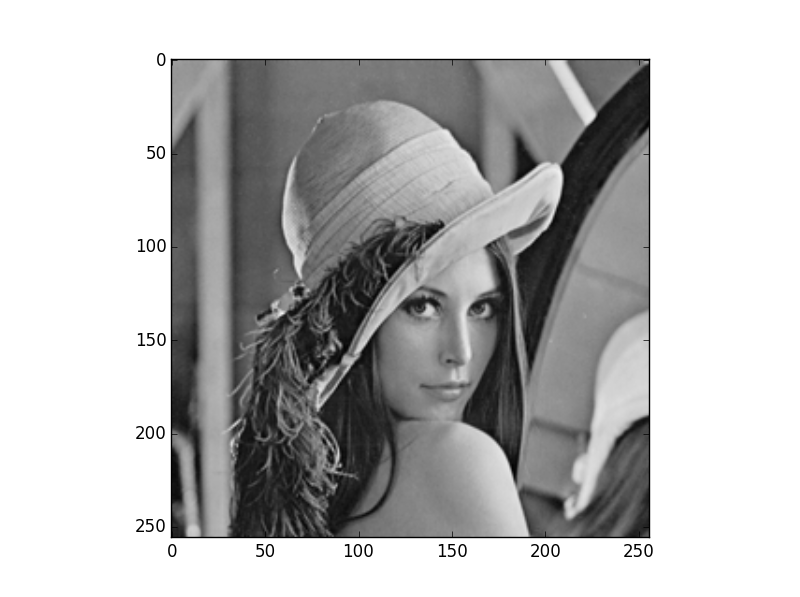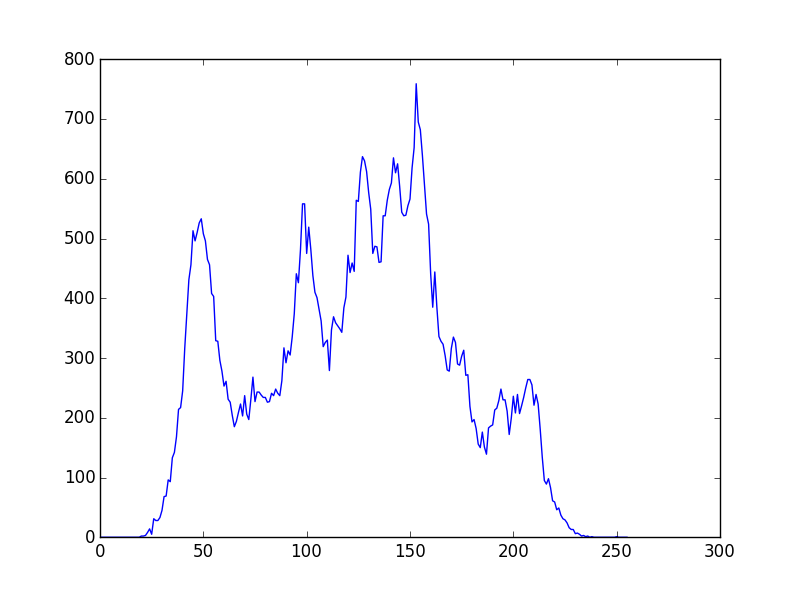# [PYTHON] How to use numpy.vectorize

### Overview

I'm addicted to how to use the python scientific calculation module numpy function vectorize, so a memo for myself By the way, this time I posted this article using the histogram creation of the input image as an example in image processing.

## Purpose of numpy.vectorize

Vectorize is a function that transforms a python function so that a list can be inserted into a function that takes a value as an argument. Each value of the input array will be calculated as an argument, and the return value will be vectorized.

## How to use numpy.vectorize

First, prepare a function whose return value is not a list.

#### `myfunc.py`

``````
def myfunc(a,b):
return a+b

print myfunc("hoge","Hoge")
``````

The output looks like this:

``````"hogeHoge"
``````

Consider plunging a vector into this myfunc.

#### `myfunc.py`

``````
def myfunc(a,b):
return a+b

list = ["hoge","fuga"]

print myfunc(list,"Hoge")
``````

I want the output to look like this:

``````["hogeHoge","fugaHoge"]
``````

But in reality

``````TypeError: can only concatenate list (not "str") to list
``````

Will be. This is because it was not originally defined to take a list as an argument. However, you can get the expected output by vectorizing myfunc with numpy.vectorize.

``````vfunc=numpy.vectorize(myfunc)
print vfunc(list,"Hoge")
``````

The output is

``````["hogeHoge","fugaHoge"]
``````

The list is returned. Now the vectorization of the function has been achieved.

## Practical example

I will leave a more practical example. Create a function that creates a histogram of the input image by image processing.

``````import numpy as np
import cv2
from matplotlib import pyplot as plt

#Function for creating a histogram
def img_hist(src,bins_array):
x = np.where(src==bins_array,1,0)
count = np.count_nonzero(x)
return count
#Vectorization
vhist = np.vectorize(img_hist)
vhist.excluded.add(0) #The 0th argument is passed to the function as it is as a fixed vector

#Prepare source file and histogram bin
bins_array = np.arange(256)

#Create a histogram
hist_array=vhist(src,bins_array)

#Visualize the histogram
plt.plot(bins_array,hist_array)
plt.show()
``````

The input image is a classic oneAs a result, the following histogram is obtained.## Summary

• I was able to organize how to use numpy.vectorize.
• It is quite convenient and should be applicable in various ways. You can program without using for loops as much as possible.